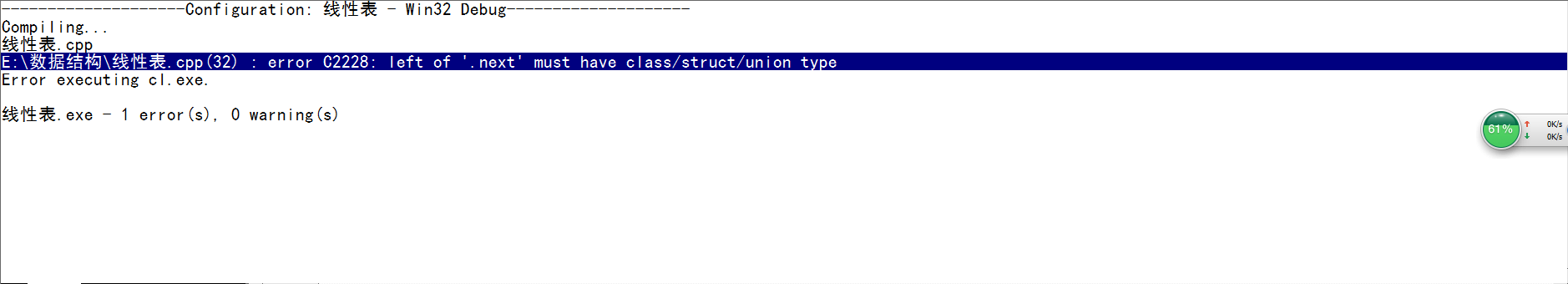``````
#include<iostream>
#include<conio.h>
#include<string.h>
#define OK 1
#define MAXSIZE 100
using namespace std;
typedef  int Status;

typedef  struct
{
char name;
float price;
}Book;
typedef Book ElemType;

typedef  struct LNode
{
ElemType data;
struct LNode *next;

int main()
{

LNode *L=new LNode();
LNode *LL;
*L.next=LL;

getch();

return 0;
}

``````1个回答

``````(*L).next=LL;

int a;
int * p = &a;
a = 10;
a = 20;
*p++; //这是 *(p++)而不是(*p)++
printf("%d", *p); //20，如果是 (*p)++，输出应该是11
``````